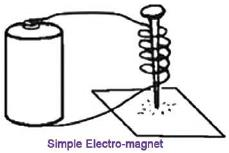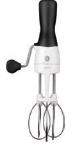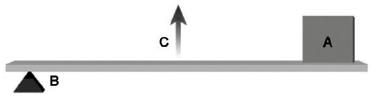Courses

# Olympiad Test: Work, Force And Energy -1

## 20 Questions MCQ Test Science Olympiad Class 4 | Olympiad Test: Work, Force And Energy -1

Description
This mock test of Olympiad Test: Work, Force And Energy -1 for Class 4 helps you for every Class 4 entrance exam. This contains 20 Multiple Choice Questions for Class 4 Olympiad Test: Work, Force And Energy -1 (mcq) to study with solutions a complete question bank. The solved questions answers in this Olympiad Test: Work, Force And Energy -1 quiz give you a good mix of easy questions and tough questions. Class 4 students definitely take this Olympiad Test: Work, Force And Energy -1 exercise for a better result in the exam. You can find other Olympiad Test: Work, Force And Energy -1 extra questions, long questions & short questions for Class 4 on EduRev as well by searching above.
QUESTION: 1

### Choose the wrong statement among followings

Solution:

Acoustics is the interdisciplinary science that deals with the study of all mechanical waves in gases, liquids, and solids including vibration, sound, ultrasound and infrasound.

QUESTION: 2

Solution:
QUESTION: 3

### The following is a diagram of a simple electromagnet.Q. How could this electromagnet be made stronger?

Solution:
QUESTION: 4

A _________  jet travels faster than sound.

Solution:
QUESTION: 5

Why we sprinkle talcum powder on a carom board?

Solution:
QUESTION: 6

Look at the following picture carefully. First identify the picture. The below machine is a combination of two simple machines. Which simple machines combine to make it?Solution:
QUESTION: 7

Electricity traveling through a wire is an example of

Solution:
QUESTION: 8

Rahul is applying force on a moving object in the same direction, the speed of the object:

Solution:
QUESTION: 9

When we throw a ball into the air, it falls back. The force responsible for this is:

Solution:
QUESTION: 10

In which of these activities, the sense of hearing is least important?

Solution:
QUESTION: 11

Two girls playing table tennis. First girl hit the ball over the net and at a Point say ‘T’, the other girl hit it back with a lot of force.
What would happen at point ‘T’?
1. The force caused a change in the size of the ball.
2. The force caused a change in the direction of the ball.
3. The force caused the ball to stop moving.

Solution:
QUESTION: 12

Air pressure is measured with a(n)

Solution:
QUESTION: 13

These products of nature are limited. Resources such as coal and oil are________________ , which means that when they are gone, nature cannot make them again. It is very important to limit their use and find other resources to replace them.

Solution:
QUESTION: 14

The _______________ works by using fuel derived from living and dead biological organisms.

Solution:
QUESTION: 15

Look at the lever below.Q. Which object has the similar working principle as the above leves.

Solution:
QUESTION: 16

While walking, some or all of the following types of energy and work are involved:

Solution:
QUESTION: 17

What happens to the total energy of a moving object if all the applied forces are conserved?

Solution:
QUESTION: 18

Mark the action in which work is being done:

Solution:
QUESTION: 19

As defined in physics, work is done:

Solution:
QUESTION: 20

If the resultant force acting on a body of constant mass is zero, the body’s momentum is:

Solution: